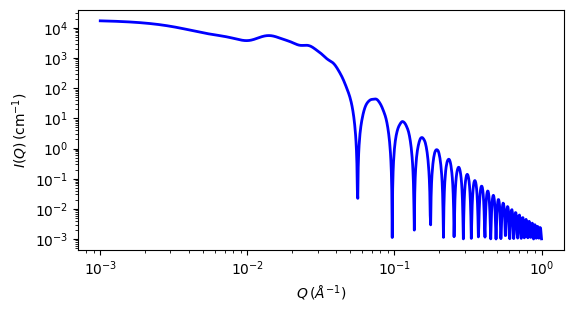# linear_pearls

Linear pearls model of scattering from spherical pearls.

Parameter

Description

Units

Default value

scale

Scale factor or Volume fraction

None

1

background

Source background

cm-1

0.001

80

edge_sep

Length of the string segment - surface to surface

350

num_pearls

Number of the pearls

None

3

sld

SLD of the pearl spheres

10-6-2

1

sld_solvent

SLD of the solvent

10-6-2

6.3

The returned value is scaled to units of cm-1 sr-1, absolute scale.

This model provides the form factor for $$N$$ spherical pearls of radius $$R$$ linearly joined by short strings (or segment length or edge separation) $$l$$ $$(= A - 2R)$$. $$A$$ is the center-to-center pearl separation distance. The thickness of each string is assumed to be negligible.

Definition

The output of the scattering intensity function for the linear_pearls model is given by (Dobrynin, 1996)

$P(Q) = \frac{\text{scale}}{V}\left[ m_{p}^2 \left(N+2\sum_{n-1}^{N-1}(N-n)\frac{\sin(qnl)}{qnl}\right) \left( 3\frac{\sin(qR)-qR\cos(qR)}{(qr)^3}\right)^2\right]$

where the mass $$m_p$$ is $$(SLD_{pearl}-SLD_{solvent})*(volume\ of\ N\ pearls)$$. V is the total volume.

The 2D scattering intensity is the same as P(q) above, regardless of the orientation of the q vector.Fig. 81 1D plot corresponding to the default parameters of the model.

Source

linear_pearls.py $$\ \star\$$ linear_pearls.c $$\ \star\$$ sas_3j1x_x.c

References

1. A V Dobrynin, M Rubinstein and S P Obukhov, Macromol., 29 (1996) 2974-2979

Authorship and Verification

• Author: